# An alternative proof of Nyblom’s results and a generalisation

A. David Christopher
Notes on Number Theory and Discrete Mathematics
Print ISSN 1310–5132, Online ISSN 2367–8275
Volume 26, 2020, Number 2, Pages 116—126
DOI: 10.7546/nntdm.2020.26.2.116-126

## Details

### Authors and affiliations

A. David ChristopherDepartment of Mathematics, The American College

### Abstract

Let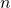be a positive integer and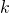be a non-negative integer. We defineand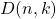to be the number of wayscan be expressed as a difference of two elements from the sequence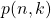. Nyblom found closed expressions forand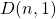in terms of some restricted number-of-divisors functions. Here we re-establish these two results of Nyblom in a relatively simple way. Along with the other interpretations for, an expression foris presented in terms of restricted form ofand. Also we consider another function due to Nyblom, denoted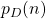, which counts the number of partitions ofwith parts in arithmetic progression having common difference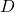. Nyblom and Evan found a simple expression for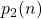and putin terms of a divisor-counting functions when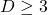. Here we re-establish Nyblom’s expression for, and find equinumerous expressions forwhen. Finally, we present the following generalised version of: given a set of positive integers say,, we denote by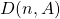, the number of wayscan be written as a difference of two elements from the set. And we expressin terms of partition enumerations when some restrictions are imposed upon the elements of. We close with the hint that, boundedness oftogether with the divergence of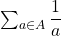disproves Erdős arithmetic progression conjecture.

### Keywords

• Square numbers
• Triangular numbers
• Representation as difference of integers

• 11A67
• 11B34

### References

1. Cook, R., & Sharp, D. (1995). Sums of arithmetic progressions, Fibonacci Quart., 33, 218–221.
2. Christopher, A. D. (2015). Partitions with Fixed Number of Sizes, J. Integer Seq., 18, Article 15.11.5.
3. Mason, T. E. (1912). On the representation of an integer as the sum of consecutive integers, Amer. Math. Monthly, 19 (3), 46–50.
4. Munagi, A. O. (2010). Combinatorics of integer partitions in arithmetic progression, Integers, 10, 73–82.
5. Nyblom, M. A. (2001). On the Representation of the Integers as a Difference of
non-consecutive Triangular numbers, Fibonacci Quart., 39 (3), 256–263.
6. Nyblom, M. A. (2002). On the Representation of the Integers as a Difference of Squares, Fibonacci Quart., 40 (3), 243–246.
7. Nyblom, M. A., & Evans, C. (2003). On the enumeration of partitions with summands in arithmetic progression, Australas. J. Combin., 28, 149–159.

## Cite this paper

Christopher, A. D. (2020). An alternative proof of Nyblom’s results and a generalisation. Notes on Number Theory and Discrete Mathematics, 26 (2), 116-126, doi: 10.7546/nntdm.2020.26.2.116-126.